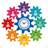# Time and WorkQuantitative Aptitude Questions and Answers section on “Time and Work” with solution and explanation for competitive examinations such as CAT, MBA, SSC, Bank PO, Bank Clerical and other examinations.

# Ronald and Elan are working on an assignment. Ronald takes 6 hours to type 32 pages on a computer, while Elan takes 5 hours to type 40 pages. How much time will they take working together on two different computers to type an assignment of 110 pages ?

Ronald and Elan are working on an assignment. Ronald takes 6 hours to type 32 pages on a computer, while Elan takes 5 hours to type 40 pages. How much time will they take working together on two different computers to type an assignment of 110 pages ? [A]7 hours 30 minutes [B]8 hours [C]8 ..

# A and B can do a piece of work in 36 days, B and C can do a it in 60 days, A and C can do it in 45 days. C alone can do it in:

A and B can do a piece of work in 36 days, B and C can do a it in 60 days, A and C can do it in 45 days. C alone can do it in : [A]90 days [B]120 days [C]150 days [D]180 days Show Answer 180 days (A + B)’s 1 day’s ..

# A and B can do a piece of work in 10 days. B and C can do it in 12 days. A and C can do it in 15 days. How long will A take to do it alone?

A and B can do a piece of work in 10 days. B and C can do it in 12 days. A and C can do it in 15 days. How long will A take to do it alone? [A]20 days [B]24 days [C]30 days [D]40 days Show Answer 24 days (A + B)’s 1 ..

# A can do a piece of work in 12 days, and B in 15 days. They work together for 5 days and then B left. The days taken A to finish the remaining work is :

A can do a piece of work in 12 days, and B in 15 days. They work together for 5 days and then B left. The days taken A to finish the remaining work is : [A]3 days [B]5 days [C]10 days [D]121 days Show Answer 3 days Work done by A and B in ..

# If A and B together can complete a piece of work in 20 days, B and C in 10 days and C and A in 12 days, then A, B and C together can complete the same work in :

If A and B together can complete a piece of work in 20 days, B and C in 10 days and C and A in 12 days, then A, B and C together can complete the same work in : [A]$latex 4\frac{2}{7}\ days&s=1$ [B]$latex \frac{7}{60}\ days&s=1$ [C]$latex 8\frac{4}{7}\ days&s=1$ [D]$latex 30\ days$ Show Answer \$latex ..